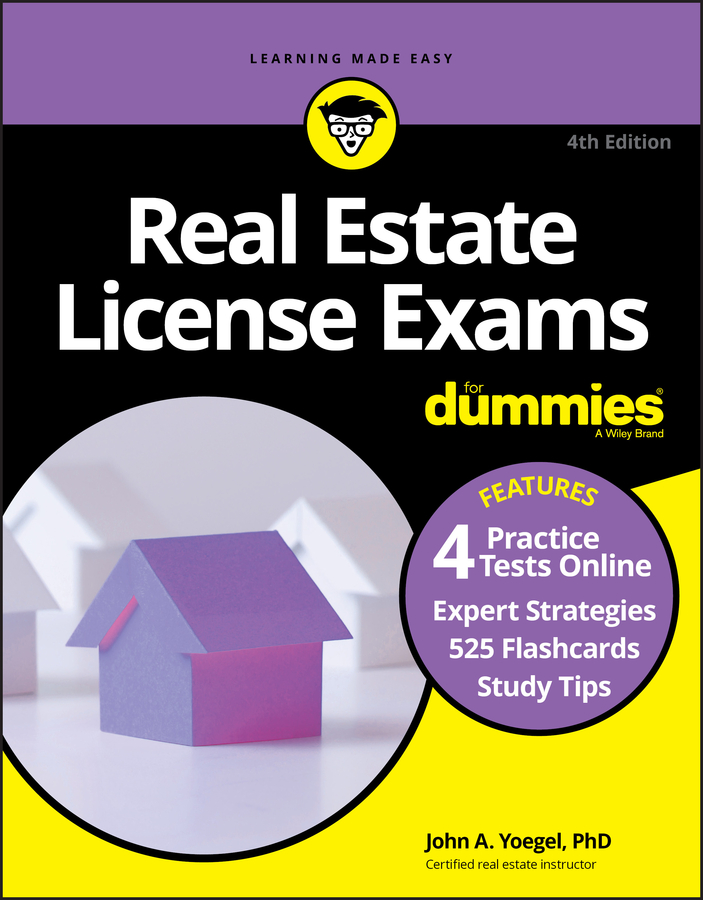##### Real Estate License Exams For Dummies with Online Practice TestsThe most popular type of loan that buyers use to purchase homes is an amortized loan, or direct reduction loan. The Real Estate License Exam will expect you to answer some math questions. The primary feature of an amortized loan is that at the end of the loan term, the loan is completely paid off. In other words, the borrower has completely paid the interest and principal of the loan.

The monthly payments can be looked up in mortgage tables, which show you amortization (or payoff) rates for various percentage interest rates over a variety of loan terms in increments of \$1,000 of loan amounts.

But if you want to want to know the amortization rate for \$1,000 at 6 percent interest for a 25-year mortgage, you can look it up in the table, and you’ll discover that the factor is \$6.44 per month. That factor is the amount of money it takes per month to pay off principal and interest on a \$1,000 loan over a period of 25 years.

So if you’re borrowing \$150,000, you simply drop three zeroes, and you get 150. Multiply that times the factor you looked up and see what you get.

150 x 6.44 = \$966

The \$966 from the equation is the monthly payment it takes to amortize a fixed-rate loan (pay off principal and interest at the same time) of \$150,000 at 6 percent for 25 years.

One other thing that you definitely need to note is that mortgage loan interest rates always are quoted on an annual basis. So the interest is a certain percent a year. In the event that an exam question wants to know the monthly rate all you do is divide the annual rate by 12.

## How to find the monthly payment when you’re given a mortgage factor

You borrow \$125,000 for 20 years at an interest rate of 7 percent. The mortgage factor is \$7.75. What is the monthly payment necessary to amortize this loan?

\$125,000 ÷\$1,000 = 125

125 x \$7.75 = \$968.75

Remember that the mortgage factor is the amount of money needed on a monthly basis to amortize on a \$1,000 loan at a given interest rate over a given period of time.

In this example, 7.75 is the amount needed to pay off a \$1,000 loan at 7 percent for 20 years. But because you’re borrowing \$125,000, you have to divide by 1,000 to find out how many units of \$1,000 are in the loan amount. Multiplying this amount by the factor gives you the answer.

## How to figure the first months interest

You borrow \$130,000 for 25 years at 7.5 percent interest in an amortized loan. How much interest do you pay in the first month of the loan?

1. \$130,000 x 0.075 (7.5 percent) = \$9,750 first year’s interest

2. \$9,750 ÷12 months = \$812.50 first month’s interest

Remember that you’re always paying interest on the unpaid balance of the loan and interest rates always are quoted on an annual basis. So in the first month, you’re paying interest on the entire unpaid balance of \$130,000 at 7.75 percent interest. The first calculation gives you the total interest for the year.

The second one gives you the first month’s interest. Note that this calculation works only for the first month, because after that, you’ve already paid off a portion of the principal, so now you’re paying interest on a lower principal.

## How to find the principal balance owed after the first payment

You borrow \$130,000 for 25 years at 7.5 percent interest in an amortized loan. The monthly payment is \$959.40. How much of the principal do you owe after you’ve made the first payment?

1. \$130,000 x 0.075 (7.5 percent) = \$9,750 first year’s interest

2. \$9,750 ÷12 months = \$812.50 first month’s interest

3. \$959.40 (principal and interest payment) – \$812.50 (interest) = \$146.90 principal

4. \$130,000 (original principal) – \$146.90 (first month’s principal) = \$129,853.10 principal owed after the first month’s payment

In Step 1, you find the total interest due for one year. Remember the mortgage interest rate is an annual rate. In Step 2, you find out the first month’s interest by dividing by 12. In case you’re wondering, this step works only for the first month in any 12-month period. In Step 3, you find out how much principal is contained in that first month’s payment.

You’re given the total monthly payment, which is made up of principal and interest. If you take away the interest, you’re left with the amount of principal you’re paying that month. In Step 4, you subtract the first month’s principal payment from the original amount of the loan, and you’re left with the remaining balance of the principal.

## How to find the total interest on the loan

Another type of exam problem that sometimes gives people a hard time is the calculation of total interest paid over the life of the loan.

You borrow \$100,000 for 30 years at 8 percent interest. It’s an amortized loan, and your monthly payments are \$734. How much interest will you pay over the life of the loan?

1. \$734 x 360 (12 months x 30 years) = \$264,240 total payments of principal and interest over the life of the loan.

2. \$264,240 (total principal and interest) – \$100,000 (loan principal) = \$164,240 interest over the life of the loan.

In Step 1, you calculate everything you pay over the life of the loan. In Step 2, you subtract the principal from the total of what you pay. Remember that the payment you make each month, and therefore the total of the payments you make, are both principal and interest, so if you subtract the principal, what you have left is the interest.